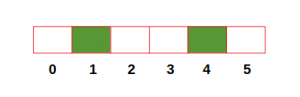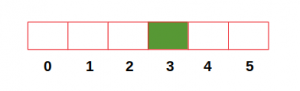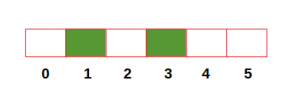GeeksforGeeks App
Open AppBrowser
Continue

# Find maximum distance between any city and station

Given the number of cities n numbered from 0 to n-1 and the cities in which stations are located, the task is to find the maximum distance between any city and its nearest station. Note that the cities with stations can be given in any order.

Examples:

```Input: numOfCities = 6, stations = [1, 4]
Output: 1

Input: numOfCities = 6, stations = 
Output: 3

Input: numOfCities = 6, stations = [3, 1]
Output: 2```
• The below figure represents the first example containing 6 cities and the cities with stations highlighted with green color. In this case, the farthest cities from their nearest stations are 0, 2, 3, and 5 each at a distance of 1. Hence, the maximum distance is 1.• In the second example, the farthest city from its nearest station is 0 which is at a distance of 3. Hence, the maximum distance is 3.• In the third example, the farthest city from its nearest station is 5 which is at a distance of 2. Hence, the maximum distance is 2.Approach:

There are three possible cases in this problem:

1. When the farthest city is between two stations.
2. When the farthest city is on the left side of the first station.
3. When the farthest city is on the right side of the last station.

Below is the algorithm to solve the above problem:

• Initialize a boolean array of size n (number of cities) with False. Then mark the values of cities with stations as True
• Initialize a variable dist with 0. Initialize another variable maxDist with a value that is equal to the first city with the station (used for case 2).
• Start looping through all the cities one by one.
• If the current city has a station, then assign the maximum of (dist+1)//2 and maxDist to maxDist (used for case 1). Also, assign 0 to dist.
• Else, increment dist.
• In the end, return the maximum of dist and maxDist (used for case 3).

Below is the implementation of the above approach:

## C++

 `// C++ program to calculate the maximum``// distance between any city``// and its nearest station``#include` `using` `namespace` `std;` `// Function to calculate the maximum``// distance between any city and its nearest station``int` `findMaxDistance(``int` `numOfCities,``int` `station[],``int` `n)``{``    ``// Initialize boolean list``    ``bool` `hasStation[numOfCities + 1] = {``false``};``    ` `    ``// Assign True to cities containing station``    ``for` `(``int` `city = 0; city < n; city++)``    ``{``        ``hasStation[station[city]] = ``true``;``    ``}``        ` `    ``int` `dist = 0;``    ``int` `maxDist = INT_MAX;` `    ``for``(``int` `i = 0; i < n; i++)``    ``{``        ``maxDist = min(station[i],maxDist);``    ``}` `    ``for` `(``int` `city = 0; city < numOfCities; city++)``    ``{``        ``if` `(hasStation[city] == ``true``)``        ``{``            ``maxDist = max((dist + 1) / 2, maxDist);``            ``dist = 0;``        ``}``        ``else``            ``dist += 1;``    ``}``    ``return` `max(maxDist, dist);``}` `//Driver code``int` `main()``{``    ``int` `numOfCities = 6;``    ``int` `station[] = {3, 1};``    ``int` `n = ``sizeof``(station)/``sizeof``(station);` `    ``cout << ``"Max Distance:"` `<< findMaxDistance(numOfCities,``                                                ``station, n);``}` `//This code is contributed by Mohit Kumar 29`

## Java

 `// Java program to calculate the maximum``// distance between any city``// and its nearest station``import` `java.util.*;` `class` `GFG``{` `// Function to calculate the maximum``// distance between any city and its nearest station``static` `int` `findMaxDistance(``int` `numOfCities,``                            ``int` `station[],``int` `n)``{``    ``// Initialize boolean list``    ``boolean` `hasStation[] = ``new` `boolean``[numOfCities + ``1``];``    ` `    ``// Assign True to cities containing station``    ``for` `(``int` `city = ``0``; city < n; city++)``    ``{``    ``hasStation[station[city]] = ``true``;``    ``}``        ` `    ``int` `dist = ``0``;``    ``int` `maxDist = Integer.MAX_VALUE;` `    ``for``(``int` `i = ``0``; i < n; i++)``    ``{``        ``maxDist = Math.min(station[i],maxDist);``    ``}` `    ``for` `(``int` `city = ``0``; city < numOfCities; city++)``    ``{``        ``if` `(hasStation[city] == ``true``)``        ``{``            ``maxDist = Math.max((dist + ``1``) / ``2``, maxDist);``            ``dist = ``0``;``        ``}``        ``else``            ``dist += ``1``;``    ``}``    ``return` `Math.max(maxDist, dist);``}` `//Driver code``public` `static` `void` `main(String args[])``{``    ``int` `numOfCities = ``6``;``    ``int` `station[] = {``3``, ``1``};``    ``int` `n = station.length;` `    ``System.out.println(``"Max Distance:"``+``        ``findMaxDistance(numOfCities,station, n));``}``}` `// This code is contributed by``// Surendra_Gnagwar`

## Python

 `# Python3 code to calculate the maximum``# distance between any city and its nearest station` `# Function to calculate the maximum``# distance between any city and its nearest station``def` `findMaxDistance(numOfCities, station):``    ` `    ``# Initialize boolean list``    ``hasStation ``=` `[``False``] ``*` `numOfCities``    ``# Assign True to cities containing station``    ``for` `city ``in` `station:``        ``hasStation[city] ``=` `True``        ` `    ``dist, maxDist ``=` `0``, ``min``(station)` `    ``for` `city ``in` `range``(numOfCities):``        ``if` `hasStation[city] ``=``=` `True``:``            ``maxDist ``=` `max``((dist ``+` `1``) ``/``/` `2``, maxDist)``            ``dist ``=` `0``            ` `        ``else``:``            ``dist ``+``=` `1``            ` `    ``return` `max``(maxDist, dist)``    ` `numOfCities ``=` `6``station ``=` `[``3``, ``1``]``print``(``"Max Distance:"``, findMaxDistance(numOfCities, station))`

## C#

 `// C# program to calculate the maximum``// distance between any city``// and its nearest station``using` `System;` `class` `GFG``{` `// Function to calculate the maximum``// distance between any city and its nearest station``static` `int` `findMaxDistance(``int` `numOfCities,``                            ``int` `[]station,``int` `n)``{``    ``// Initialize boolean list``    ``bool` `[]hasStation = ``new` `bool``[numOfCities + 1];``    ` `    ``// Assign True to cities containing station``    ``for` `(``int` `city = 0; city < n; city++)``    ``{``        ``hasStation[station[city]] = ``true``;``    ``}``        ` `    ``int` `dist = 0;``    ``int` `maxDist = ``int``.MaxValue;` `    ``for``(``int` `i = 0; i < n; i++)``    ``{``        ``maxDist = Math.Min(station[i],maxDist);``    ``}` `    ``for` `(``int` `city = 0; city < numOfCities; city++)``    ``{``        ``if` `(hasStation[city] == ``true``)``        ``{``            ``maxDist = Math.Max((dist + 1) / 2, maxDist);``            ``dist = 0;``        ``}``        ``else``            ``dist += 1;``    ``}``    ``return` `Math.Max(maxDist, dist);``}` `// Driver code``public` `static` `void` `Main(String []args)``{``    ``int` `numOfCities = 6;``    ``int` `[]station = {3, 1};``    ``int` `n = station.Length;` `    ``Console.WriteLine(``"Max Distance:"``+``        ``findMaxDistance(numOfCities,station, n));``}``}` `// This code has been contributed by 29AjayKumar`

## PHP

 ``

## Javascript

 `   `

Output

`Max Distance:2`

Complexity Analysis:

• Time Complexity: O(n)
• Space Complexity: O(n)

My Personal Notes arrow_drop_up